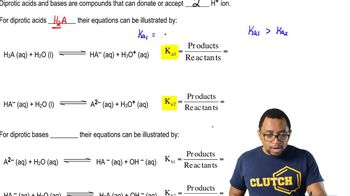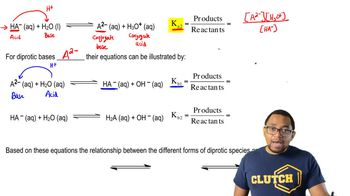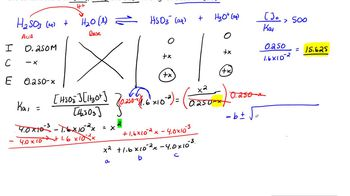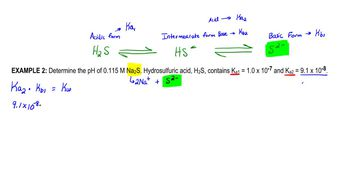Start typing, then use the up and down arrows to select an option from the list.# Diprotic Acids

Jules Bruno
190views
in this video, we'll take a look at die product acids. Now we're gonna stay here that die product acids and bases are compounds that can donate or accept to H plus ions. Now let's talk about die product acids. First, we're gonna say Die product acids. The general formula for them will be H two A. That's if you don't know what the dye product as it looks like. But you know what? The dye product as it so it has to acidic hydrogen. That's why it's H two and then a represents the nominal portion. Okay, now they're equations can be illustrated by when we're dealing with di product acids. That means we can donate H plus two times. The first H plus that we donate is associated with K one. Okay, so that's your acid association, Constant one. Then, if you're gonna give away the second acidic hydrogen that deals with K two, what you need to realize here about K one and K two is that K one will always be greater than K two. It's always easier to donate the first H plus and then much more difficult to donate the second hydrogen and in fact, they're usually different by several magnitudes. What I mean by that is a k A one. Let's say it Waas 1.3 times 10 to the negative too. You would expect your K two to be much smaller than that. Maybe like 8.5 times 10 to the negative five. I'm just making up these numbers, but I'm illustrating how they're different by several magnitudes here. This is to the negative to whereas this is to the negative five. So they're different by it. Magnitude of three here. Okay, so there's a big difference in strength. Now, if we're looking at our equilibrium equation, remember H two way this will represent our acid water. He will represent our base. Remember? Based on the Bronston Laurie idea of acids and bases, acids donate H plus bases except H plus. So now, my ass, it has become my conjugate base and water accepted in H plus. So now it's H +30 plus. So this is the conjugate acid. If we're talking about the equilibrium expression that means K one equals products overreact. INTs remember, we ignore solids and liquids. Water is a liquid, so it'll be ignored. So this would be a check a minus times H plus divided by H two Way. Now, after we've given away the first acidic hydrogen we have. This left this because there's still another H plus in it. It has the potential to donate that second one if it decides to donate that second H plus. Then we come down here to this equation. So here it is now it's already given away the first H plus. Now it's about to give away the second one. So again it's the acid water is gonna act as the base, so it's gonna give away an H plus. As a result, it becomes even more negative. So now it's a two minus. So this would be the conjugate base here. Water accepted an H plus. So now it's the conjugate acid we're talking about giving away its second acidic hydrogen. So now we're dealing with K two, and so this would be a to minus times H 30 plus, divided by H A minus. So these will be our to equilibrium expressions dealing with di protic acid, donating it's H plus ions, so give yourself a minute or two to absorb the information that we've just presented in terms of die product acids and then we'll move on to die product basis.03:4302:2304:1413:3406:41